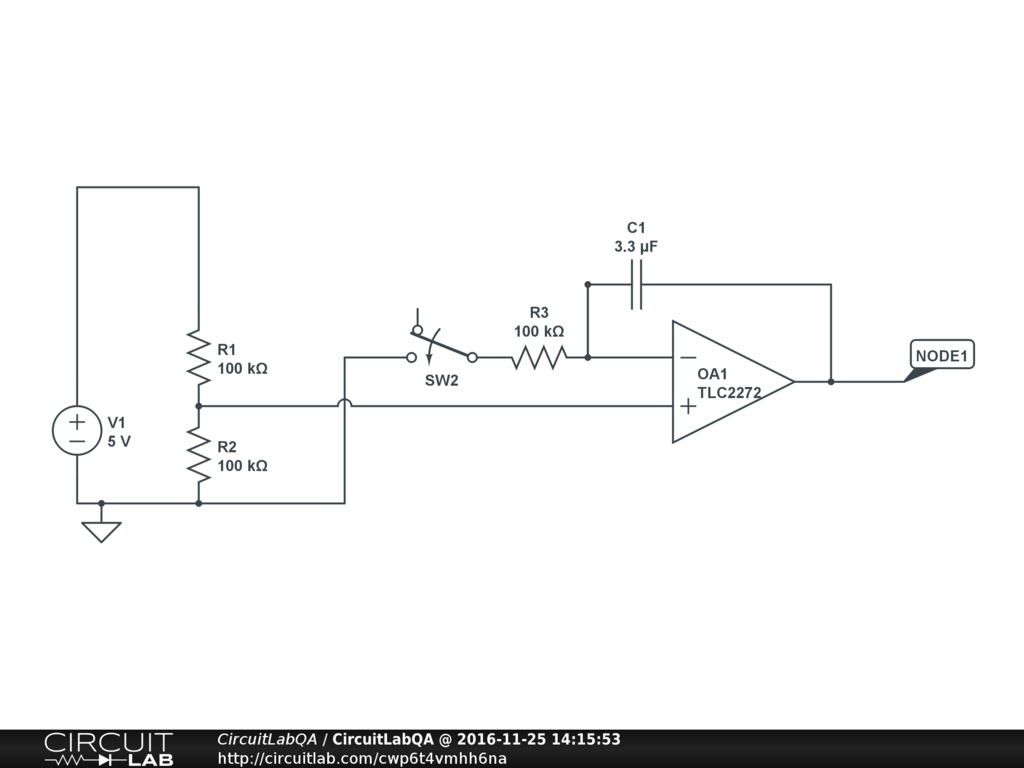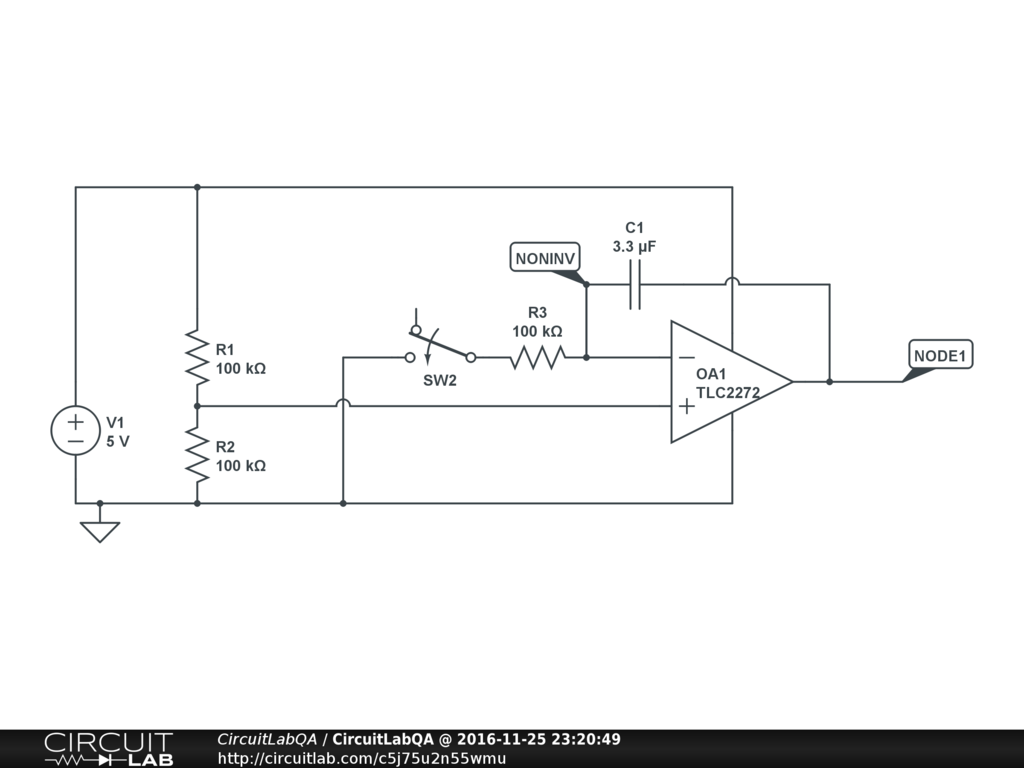## Why integrator stops integrating?

 I made this opamp integrator on breadboard:In the simulator it works, output starts at 2.5 and starts rising and rising. But on oscilloscope it stops rising after I flip switch about half a second and stays at about 5V. by vivgre78 November 25, 2016

 In real life your op-amp output can only go as high as your positive voltage rail. (Or sometimes a bit less, depending on how the output stage of the op-amp is built -- check the datasheet!) So it's going to integrate until it maxes out, but then stop. Your circuit uses R1 and R2 to make a voltage divider putting 2.5V on the non-inverting input. When the switch closes [assuming the capacitor C1 is initially discharged] you'll have a the op-amp immediately to 2.5V, since there's no voltage across the capacitor, which will momentarily make the two op-amp input equal. But that means R3 will have 2.5V across it, so $\frac {2.5 V} {100 k \Omega} = 25 \mu A$ will flow through R3. And since that current can't go into the inverting terminal of the op-amp, it will also flow through C1. This causes the voltage across the capacitor to increase at a rate of: $$Q=C V$$ $$\frac {dQ} {dt}= i = C \frac {dV} {dt}$$ $$\frac {dV} {dt} = \frac {i} {C} = \frac {25 \mu A} {3.3 \mu F} = 7.58 \frac {V} {s}$$ But once the total output voltage hits 5V -- an increase of 2.5V over its starting point -- the op-amp can't increase anymore! That happens at about $t = \frac {2.5 V} {7.58 V/s} = 0.329 s$. I copy and pasted your circuit but changed the op-amp to have voltage rails in the simulation:Run the simulation now -- it does what you expect. It's possible that nobody else will find this interesting, but what do you think happens to the voltage at the non-inverting op-amp terminal after the output saturates? I plotted it as V(NONINV) and also plotted the current through the capacitor. It turns out that after the output saturates, R3 and C1 form a simple RC circuit, and the voltage V(NONINV) exponentially approaches ground. Pretty cool! +1 vote by mrobbins November 25, 2016

CircuitLab's Q&A site is a FREE questions and answers forum for electronics and electrical engineering students, hobbyists, and professionals.

We encourage you to use our built-in schematic & simulation software to add more detail to your questions and answers.

Acceptable Questions:

• Concept or theory questions
• Practical engineering questions
• “Homework” questions
• Software/hardware intersection
• Best practices
• Design choices & component selection
• Troubleshooting

Unacceptable Questions:

• Non-English language content
• Non-question discussion
• Non-electronics questions
• Vendor-specific topics
• Pure software questions
• CircuitLab software support

Please respect that there are both seasoned experts and total newbies here: please be nice, be constructive, and be specific!